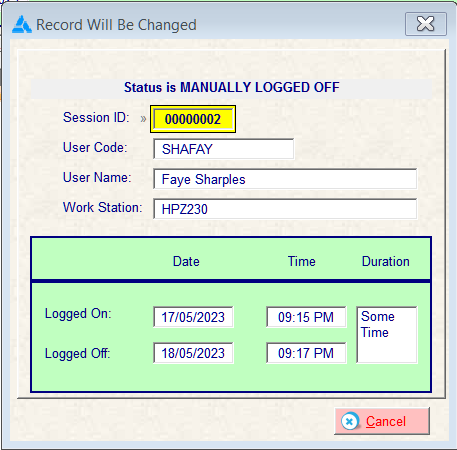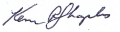# How to calculate the time lapsed between two dates & times?

The exercise records the date and time a user logs onto a program, and at a later time logs off. The exercise is to measure the time lapsed.
The lapsed time may extend over multiple days, and needs to return days, hours and minutes. Any assistance or direction would be appreciated.I haven’t tested this at all, but something like this seems like what you’re looking for.

``````TIME:Tick   EQUATE(  1)
TIME:Second EQUATE(100 * TIME:Tick)
TIME:Minute EQUATE( 60 * TIME:Second)
TIME:Hour   EQUATE( 60 * TIME:Minute)
TIME:Day    EQUATE( 24 * TIME:Hour  )

Dates.Span PROCEDURE(LONG xDateA, LONG xTimeA, LONG xDateB, LONG xTimeB, *LONG xOutSpanDays, *LONG xOutSpanTime)
FirstDate  LONG,AUTO
FirstTime  LONG,AUTO
SecondDate LONG,AUTO
SecondTime LONG,AUTO

CODE
IF (xDateA < xDateB) OR (xDateA = xDateB AND xTimeA < xTimeB)
FirstDate  = xDateA
FirstTime  = xTimeA
SecondDate = xDateB
SecondTime = xTimeB
ELSE
FirstDate  = xDateB
FirstTime  = xTimeB
SecondDate = xDateA
SecondTime = xTimeA
END

xOutSpanDays = SecondDate - FirstDate

IF FirstTime <= SecondTime
xOutSpanTime = SecondTime - FirstTime
ELSE
xOutSpanDays -= 1
xOutSpanTime  =  FirstTime - ( SecondTime - TIME:Day )
END
``````
2 Likes

You have the Duration class that was done as part of a series of Clarion Live Webinars.

Open the Clarion Live Search and type Duration. Part 1 was #491 on Dec 7 2018, Part 2 was #495 on Jan 4 2019, Part 3 was #497 on Jan 18.

1 Like

You should read the help on Clarion Standard Date so you understand a Date in a LONG is stored as the number of Days since 12/28/1800. To get Days between dates you can simply subtract the 2 dates. It harder to use human understandable forms for Day, Month, Year. For that use FORMAT(, @d#) or functions Day(), Month(), Year(), Date().

Also read about Clarion Standard Time so you understand a Time in a LONG is stored as the number of Hundredths of Seconds since midnight … Plus 1 … that +1 is important.

``````TimeHMS  PROCEDURE(SHORT H, SHORT M, SHORT S=0, SHORT Hundredths=0)!,LONG
!This allows out of range HMS values, e.g. you can pass 90 minutes. This can produce an invalid time beyond 23:59:59.
CODE
RETURN (H * 60*60*100) + |
(M *    60*100) + |
(S *       100) + |
Hundredths + 1
``````

The DATE and TIME types are different with the Internal Format as YYYYMMDD and HHMMSShh. Anytime they are used in code they are converted to Standard versions i.e. YMD becomes Days since 12/28/1800.

These can be used in code like below to split a DATE or TIME into parts. For dates you have functions for this. Time does not have these so this type of function can be used, or FORMAT(,@T05) that’s HHMMSS.

``````DateSplit        PROCEDURE(LONG Date2Split, *? OutMonth, *? OutDay, *? OutYear)
!==============================================================================
DateSplit PROCEDURE(LONG D, *? OutMonth, *? OutDay, *? OutYear)
!This is much faster than calling MONTH() DAY() YEAR()
D1 DATE
DG GROUP, OVER(D1)
D BYTE
M BYTE
Y USHORT
END
CODE
D1=D
OutMonth=DG.M
OutDay=DG.D
OutYear=DG.Y
RETURN
!--------------------------------------------------------------------

TimeSplit PROCEDURE(LONG T, *? OutHour, *? OutMin, <*? OutSec>, <*? OutHund>)
!This is faster than using HOUR() MINUTE() SECOND()
T1 TIME
TG GROUP, OVER(T1)
F BYTE
S BYTE
M BYTE
H BYTE
END
CODE
T1=T
OutHour=TG.H
OutMin =TG.M
IF ~OMITTED(OutSec) THEN OutSec =TG.S.
IF ~OMITTED(OutHund) THEN OutHund=TG.F.
RETURN

``````

Many thanks for your detailed response.Likely irrelevant and late, but I do not see it explicitly stated that topspeed files are being used. For SQL users, calculating an interval with Clarion code is probably not necessary.

Here is something I use on signing out of a time clock. It’s a bit different and doesn’t care much about seconds.

First when clicking the signout button:
HOU:SIGNOUT=clock()
HOU:OUTDATE=today()
do figmins
HOU:TOTAL_HRS=(minutes * 6000) + 1

DISPLAY

figmins routine
set:round_time=0
hou:signin=deformat(format(hou:signin,@t3),@t3)
hou:signout=deformat(format(hou:signout,@t3),@t3)

``````if hou:outdate-hou:indate > 0

!**** get time for first day
td\$ = 8640000 - hou:signin  !8640000 = # of 100ths of a sec in one day
minutes = round(td\$/6000,1) !*** num of minutes

!**** get time for days inbetween day 0 and last day
if hou:outdate - hou:indate > 1
minutes = minutes + (((hou:outdate-hou:indate)-1)*1440)
.

!**** get time for last day
minutes+=round(hou:signout/6000,1)

else
!**** if the days are the same
minutes = round((hou:signout - hou:signin) / 6000,1)
minutes = round(minutes,1)
.

!***** round to nearest minutes here ***
if set:round_time>0
if minutes => SET:ROUND_TIME
rem\$ = (minutes/set:round_time) - int(minutes/SET:ROUND_TIME) !remainder of minutes
if rem\$ >= .5
minutes = (int(minutes/SET:ROUND_TIME) * SET:ROUND_TIME) + SET:ROUND_TIME  !round up
else
minutes = (int(minutes/SET:ROUND_TIME) * SET:ROUND_TIME)  !round down
.
else
minutes=SET:ROUND_TIME !if less then the round time is equal to the round time
.
.
exit
``````

set:round_time is a local byte variable and I think its to round to the nearest 5 minutes or 15 minutes or whatever you change it to. Here I think it’s just a zero.

Hope that helps someone.